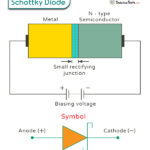Home / Physics / Applied Force

# Applied Force

## What is Applied Force

An applied force is a contact force that is applied to an object by external means. As a result of applied force, the object either moves or deforms. The object generally moves in the direction of the applied force. Suppose it is lifted from the ground using a pulley. In that case, the motion of the object is opposite to the direction of the applied force.

## Examples of Applied Force

Here are some examples of the applied force.

• Pulling a bucket of water from a well
• Rotating a doorknob by human hand
• Lifting an object from the floor
• Checking the weight of the person (This is a practice way to measure the applied force)
• Pushing a box on a floor

## Applied Force Formula: How to Find the Applied Force

The applied force can be determined using Newton’s laws of motion and the force balance equation in different situations.

### Applied Force Equation

Suppose an object of mass m is pushed through a distance such that its acceleration is a. Then, the applied force is given by,

FA = ma

Friction

Now, if the object is moving on a surface where there is a friction force f, then,

ma = FA – f

Or, FA = ma + f

Gravity

If a string suspends the object, then its weight W is the only force acting on it. The applied force is given by,

FA = W = mg

Inclined Plane

When the object is on an inclined plane, then the component of its weight along the incline is the applied force.

FA = mg sin θ

Work Done by Applied Force

When the applied force displaces an object through a distance x, work is done.

W = FA.x

## Applied Force vs. Normal Force

The applied force is the force applied to the object to either displace it or change its shape. The normal force is the force exerted on the object by its support (e.g., table and floor). The two of them can co-exist simultaneously and act on the same object.Schottky DiodeBipolar Junction TransistorHalf-Wave RectifierFull Wave Rectifier